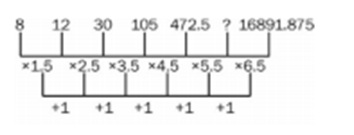# RRB ALP 2018 Practice Test Papers | Arithmetic Questions (Day-3)

Dear Aspirants, Here we have given the Important RRB ALP & Technicians Exam 2018 Practice Test Papers. Candidates those who are preparing for RRB ALP 2018 can practice these Arithmetic Questions to get more confidence to Crack RRB 2018 Examination.

[WpProQuiz 1454]

Click “Start Quiz” to attend these Questions and view Explanation

1. If a & b are whole numbers such that ab = 361 then (a+1)b-1 =?

(A) 10

(B) 30

(C) 20

(D) 11

1. In an examination, it is required to get 64% of the aggregate marks to pass. A student gets 440 marks and is declared failed by 9% marks. What are the maximum aggregate marks can a student get?

(A) 850

(B) 780

(C) 900

(D) 800

1. Giridhar purchased 100 sarees at the rate of Rs. 450 per piece. While selling, he offered 10% discount on labelled price and earned a profit of 20%. What was the labelled price of each saree?

(A) Rs. 540

(B) Rs.600

(C) Rs. 590

(D) Rs. 650

1. The sum of two numbers is 23 and the difference is 5. What is the sum of their squares?

(A) 277

(B) 291

(C) 349

(D) 554

1. How many different words can be formed by using the letters of the word ALLAHABAD so that the vowels are always together?

(A) 512

(B) 360

(C) 396

(D) 420

1. The HCF of two numbers in the ratio 2: 7 is 23. Find their LCM.

(A) 322

(B) 46

(C) 151

(D) 197

7.If the sum and product of the roots of a quadratic equation are 8 and 15, then the quadratic equation is

(A) x2 – 8x – 15 = 0

(B) x2 + 8x + 15 = 0

(C) x2 – 8x + 15 = 0

(D) x2 + 8x – 15 = 0

1. A sphere and a right circular cylinder have equal volume and radii. What is the ratio of the height of the cylinder to the radius?

(A) 3: 4

(B) 4: 3

(C) 9: 16

(D) √3: √4

1. Select the option that will replace the question mark (?) in the given series to make it continuous.

8, 12, 30, 105, 472.5, ? , 16891.875

(A) 2598.75

(B) 2597.25

(C) 2598.25

(D) 2545.25

1. What should be placed in place of x so that 23567×32 will be divisible by 11?

(A) 4

(B) 6

(C) 5

(D) 7

The given expression is:  ab = 361

ab = 192

Comparing both sides, we get a = 19 & b = 2

Value of (a+1)b-1=( 19+1)2-1= (20)1= 20.

Marks obtained by a student = (64% – 9%) of maximum aggregate marks = 55% of the maximum aggregate marks

55% of aggregate marks = 440

Aggregate marks = 440/55*100= 800

Discount = 10% (Given)

Therefore, S.P. = 90% of M.P ….(1)

Profit = 20% (Given)

Therefore, S.P. = 120% of C.P…..(2)

By combining (1) and (2), we get:

90% of M.P. = 120% of C.P.

M.P. = 4/3 of C.P. = 4/3 × 450 = 600

Let the first number be x Second number = 23 – x

According to the question, x – (23 – x) = 5 x – 23 + x = 5 2x = 28 x = 14

First number = 14

Second number = 9

Sum of the squares of numbers = 142 + 92 = 196 + 81 = 277

There are 4 vowels and 5 consonants in the word ALLAHABAD.

Let’s consider 4 vowels to be 1 letter.

Now, we have 5 + 1 = 6 letters, and these 6 letters can be arranged in 6!/2! = 360 ways. (Note that the consonant L is there twice in the word.)

Also, the 4 vowels can be arranged among themselves in 4!/4! ways.

Therefore, the total number of words that can be formed is 360 × 1 = 360.

Since the numbers are in the ratio 2: 7 and HCF is 23, the required LCM = 2 × 7 × 23 = 322

Equation with roots α and β is x2 – (α + β) x + αβ = 0. Hence, the required equation is x2 – 8x + 15 = 0.

Let the radius be r and height of the cylinder is h.

4/3 πr3= πr2h

4/3 r=h

h/r =4/3472.5 × 5.5 = 2598.75

A number is divisible by 11 if the difference between the sum of numbers at even places and the sum of numbers at odd places is either 0 or divisible by 11.

The difference of the sum of numbers at even places and odd places = 2 + 5 + 7 + 3 – (3 + 6 + x + 2)

= 17 – 11 – x

= 6 – x

6 – x is zero, when x = 6

RRB ALP 2018 Practice Test Papers | Arithmetic Questions (Day-1)

RRB ALP 2018 Practice Test Papers | Arithmetic Questions (Day-2)